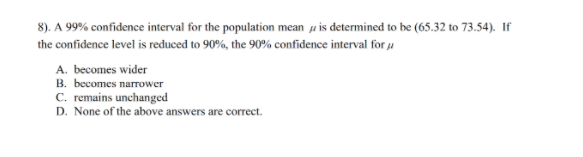# Question thank. you for help 8). A 99% confidence interval for the population mean y is determined to be (65.32 to 73.54). If the confidence level is reduced to 90%, the 90% confidence interval for å A. becomes wider B. becomes narrower C. remains unchanged D. None of the above answers are correct.4OXUNE The Asker · Probability and Statistics

thank. you for helpTranscribed Image Text: 8). A 99% confidence interval for the population mean y is determined to be (65.32 to 73.54). If the confidence level is reduced to 90%, the 90% confidence interval for å A. becomes wider B. becomes narrower C. remains unchanged D. None of the above answers are correct.
More
Transcribed Image Text: 8). A 99% confidence interval for the population mean y is determined to be (65.32 to 73.54). If the confidence level is reduced to 90%, the 90% confidence interval for å A. becomes wider B. becomes narrower C. remains unchanged D. None of the above answers are correct.